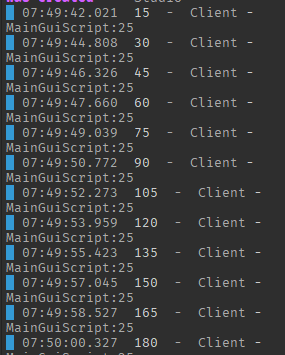# How do i know if how much value added to an intvalue roblox

so i have this script! but it seems it only prints 15 then to further but i want it to print how much value it got added. How do i achieve that

``````Stomach.Changed:Connect(function(String)

print(String)

end)
``````you would need a reference point of the initial value

``````local InitialString = Stomach.Value

Stomach.Changed:Connect(function(String)

print(InitialString)
print(String)

InitialString=String
--do calculations
end)
``````
2 Likes
``````local intvalue = -- path to your int value

intvalue.Value += 1
end

intvalue:GetPropertyChangedSignal("Value"):Connect(function()
print(intvalue.Value)
end)
``````

Use :PropertyChangedSignal as it’s way more accurate because it doesn’t have to connect.

Combining ideas from the previous posts,

``````local stomach
local lastValue = stomach.Value

stomach:GetPropertyChangedSignal("Value"):Connect(function()
local newValue = stomach.Value
local difference = newValue - lastValue
print(difference)

lastValue = newValue
end)
``````

The previous value of `stomach` is logged every time the `value` property changes, allowing you to compare the new and old values. Subtracting the previous value from the new value will tell you how much the value added/subtracted.

3 Likes

Thank you so much for this man

This topic was automatically closed 14 days after the last reply. New replies are no longer allowed.